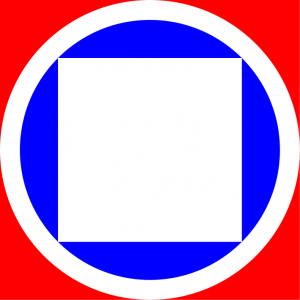01/2/18

# Visualizing the Inscribed Circle and Square Puzzle

Recently, I watched a cool mind your decsions video on an inscribed circle and rectangle puzzle. In the video they showed a diagram that was not scale. I wanted to get a sense of how these differently shaped areas will match.

There was a cool ratio between the outer and inner circle radii that is expressed as

$$\frac{R}{r}=\sqrt{\frac{\pi-2}{4-\pi}}$$.

I used Compose.jl to rapidly do that.

using Compose
set_default_graphic_size(20cm, 20cm)
ϕ=sqrt((pi -2)/(4-pi))
R=10
r=R/ϕ
ctx=context(units=UnitBox(-10, -10, 20, 20))
composition = compose(ctx,
(ctx, rectangle(-r/√2,-r/√2,r*√2,r*√2),fill("white")),
(ctx,circle(0,0,r),fill("blue")),
(ctx,circle(0,0,R),fill("white")),
(ctx,rectangle(-10,-10,20,20),fill("red")))
composition |> SVG("inscribed.svg")